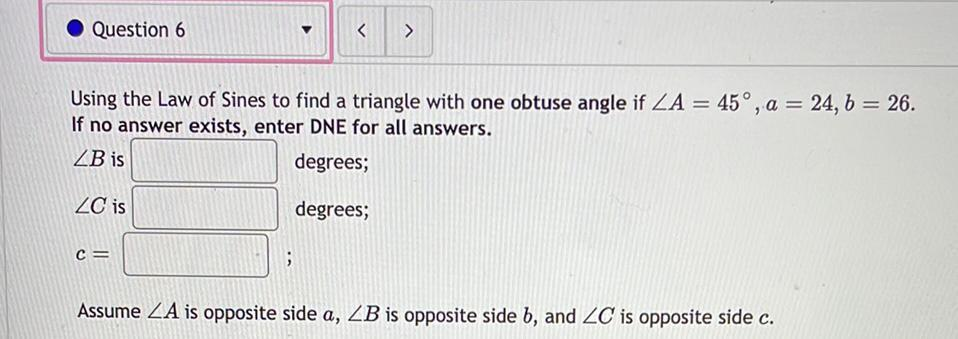Question:

# Question 6 < > Using the Law of Sines to find a triangle with one obtuse angle if ZA = 45°, a = 24, b = 26. If no answer exists,Question 6 < > Using the Law of Sines to find a triangle with one obtuse angle if ZA = 45°, a = 24, b = 26. If no answer exists, enter DNE for all answers. ZB is degrees; ZC is degrees; C= ; Assume ZA is opposite side a, ZB is opposite side b, and ZC is opposite side c.